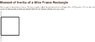# A tricky Moment of Inertia question, help needed

nikkou
[SOLVED] a tricky Moment of Inertia question, help needed...

The question is in the JPG attachment.
This is what i tried:
Obviously the side which is right on the axis has no moment of inertia so we're left with the 2 horizontal ones and the other vertical. for the two horizontal sides we've got an equation: (M*L^2)/3 and we consider it twice.
the last side is probably where i went wrong - i thought it's simply M*L^2 because it can be regarded as a point mass at distance L but that didn't work. then i tried using the parallel axis theorem but that didn't help too.
Any thoughts? I'm sure its really easy for most of you guys...
Thanx.

#### Attachments

•Untitled-1.jpg
15.7 KB · Views: 602

Homework Helper
The m given in the picture is that of the whole mass -- I hope you have taken that into account. The MI of the vertical side is equal to (m/4)a^2, as you had correctly deduced.

The MI of a rod about its end is ML^2/3, but you have written a different formula.

nikkou
Well, I did use 1/4 of the given mass for each rod. sorry for not mentioning it. and the formula i wrote is the same one you did: (ML^2)/3 . still it doesn't work...
The real answer, which i forgot to give, is: 3.97×10-3 kg*m^2
I never get it...

Homework Helper

MI = (m/4)(2a²/3 + a²) = (m/4)(5/3)a² = 39703.125 g*cm², which is your answer.

In this sort of problems, where the values are in CGS, it's better to calculate in CGS and then convert at one stroke to SI.

Last edited: